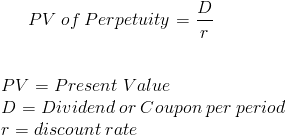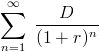Custom Search

# PV of Perpetuity

PV of Perpetuity Calculator (Click Here or Scroll Down)A perpetuity is a type of annuity that receives an infinite amount of periodic payments. An annuity is a financial instrument that pays consistent periodic payments. As with any annuity, the perpetuity value formula sums the present value of future cash flows.

Common examples of when the perpetuity value formula is used is in consols issued in the UK and preferred stocks. Preferred stocks in most circumstances receive their dividends prior to any dividends paid to common stocks and the dividends tend to be fixed, and in turn, their value can be calculated using the perpetuity formula.

The value of a perpetuity can change over time even though the payment remains the same. This occurs as the discount rate used may change. If the discount rate used lowers, the denominator of the formula lowers, and the value will increase.

It should be noted that the formula shown supposes that the cash flows per period never change.

### Example of Perpetuity Value Formula

An individual is offered a bond that pays coupon payments of \$10 per year and continues for an infinite amount of time. Assuming a 5% discount rate, the formula would be written asAfter solving, the amount expected to pay for this perpetuity would be \$200.

#### Alternative Formula

The perpetuity value formula is a simplified version of the present value formula of the future cash flows received per period. The present value or price of the perpetuity can also be written asAnother way of showing this equation isThis infinite geometric series can be simplified to dividend per period divided by the discount rate, as shown in the formula at the top of the page.

New to Finance?